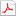검색어 입력폼

# [전자기학] 전자기학(McGrawHill)4장연습문제풀이

등록일 2002.10.30어도비 PDF (pdf) | 9페이지 | 가격 3,000원

## 목차

4.1.The value of E at P (ρ =2 ,φ =40 ◦,z =3)is given as E =100 a ρ
−200 a φ +300 a z V/m.
Determine the incremental work required to move a 20 µC charge a distance of 6 µm:

4.3.If E =120 a ρ V /m,ﬁnd the incremental amount o work done in moving a 50 µm charge a
distance of 2 mm from:

4.5.Compute the value of
P
A G ·d L for G =2 y a x with A (1 , −1 ,2)and P (2 ,1 ,2)using the path:

4.6.Let G =4 x a x +2 z a y +2 y a z .Given an initial point P (2 ,1 ,1)and a ﬁnal point Q (4 ,3 ,1),ﬁnd
G ·d L using the path:a)straight line:y =x −1,z =1;b)parabola:6 y =x 2 +2,z =1:

4.7.Repeat Problem 4.6 for G =3 xy 3 a x +2 z a y .Nowthings are di ﬀerent in that the path does
matter:
...

## 본문내용

4.1.The value of E at P (ρ =2 ,φ =40 ◦,z =3)is given as E =100 a ρ
−200 a φ +300 a z V/m.
Determine the incremental work required to move a 20 µC charge a distance of 6 µm:
a)in the direction of a ρ :The incremental work is given by dW = −q E ·d L ,where in this
case,d L =dρ a ρ =6 ×10 −6 a ρ .Thus
dW = −(20 ×10 −6 C)(100 V /m)(6 ×10 −6 m)= −12 ×10 −9 J= −12 nJ
b)in the direction of a φ :In this case d L =2 dφ a φ =6 ×10 −6 a φ ,and so
dW = −(20 ×10 −6 )( −200)(6 ×10 −6 )=2 .4 ×10 −8 J =24 nJ
c)in the direction of a z :Here,d L =dz a z =6 ×10 −6 a z ,and so
dW = −(20 ×10 −6 )(300)(6 ×10 −6 )= −3 .6 ×10 −8 J= −36 nJ
d)in the direction of E :Here,d L =6 ×10 −6 a E ,where
a E =100 a ρ

## 참고 자료

McGrawHill Electomagnetics

Hayt.. buck...